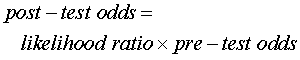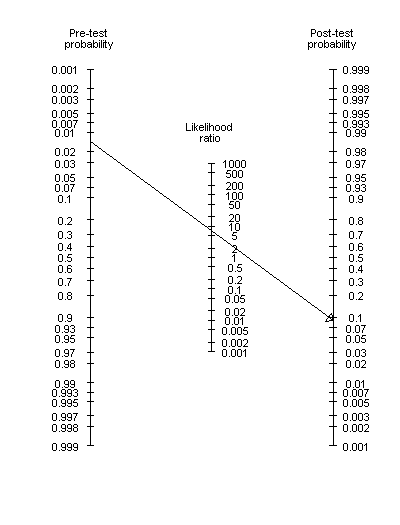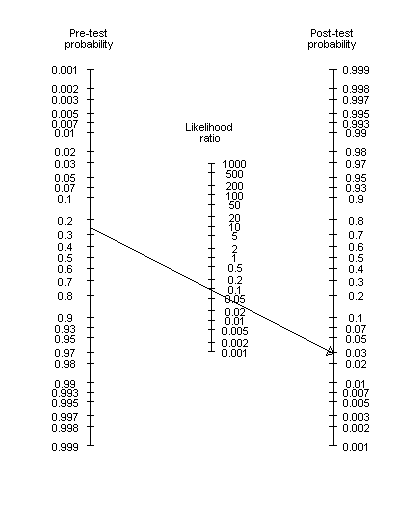StATS: What is a Fagan nomogram?

The Fagan nomogram is a graphical tool for estimating how much the result on a diagnostic test changes the probability that a patient has a disease (NEJM 1975; 293: 257). A picture of the Fagan nomogram appears below.

To use this tool, you need to provide your best estimate of the probability of the disease prior to testing. This is usually related to the prevalence of the disease, though this may be modified up or down on the basis of certain risk factors that are present in your patient pool or possibly in this particular patient. You also need to know the likelihood ratio for the diagnostic test.

With this information, draw a line connecting the pre-test probability and the likelihood ratio. Extend this line until it intersects with the post-test probability. The point of intersection is the new estimate of the probability that your patient has this disease.More details

Here are details on how the graph works and how you could construct a similar graph yourself. The principle is very much similar to a slide rule.

First, the computations involved use odds rather than ratios. If you multiply the pre-test odds by the likelihood ratio, you will get the post-test odds. And since multiplication of two numbers is equivalent to adding their logarithms, we use a log scaling for both the odds and the likelihood ratio.

The official formula is:and the Fagan nomogram uses the equivalent formulaSo although the labels on the left and right are written in terms of probability, the tick marks are spaced at the log odds. For technical reasons.we have to set the scaling of the log likelihood ratio to 1/2 that of the log odds. We also have to invert the scale for the log pre-test odds.

So if you wanted to construct this graph yourself, simply plot a range of log odds at x=+1. Plot an inverted range of log odds at x=+1. Write labels in terms of probabilities rather than odds. Then plot 1/2 of the log likelihood ratio values at x=0.

Examplehttp://jama.ama-assn.org/cgi/reprint/291/24/2990.pdf

Here are a couple examples of how to use the Fagan nomogram.

A study of an early test for developmental dysplasia of the hip (AJPH 1998; 88(2): 285-288) computed a likelihood ratio for a positive result as 7 for boys (5 for girls). The prevalence of this condition is 1.5% in boys (6% for girls). Suppose one of our patients is a boy with no special risk factors. The diagnostic test is positive. What can we say about the chances that this boy will develop hip dysplasia?The post-test probability is a bit below 10%.

Suppose this boy had a family history of hip dysplasia that would increase our pre-test probability to 25%. How much would our assessment change if we.had a negative test result?

The likelihood ratio for a negative result is 0.09 for boys (0.2 for girls) So we would draw a line connecting the pre-test probability of slightly more than 20% to a likelihood ratio about just a bit below .1This leads to a post-test probability around 3%.

A web based version of the Fagan Nomogram is available at www.cebm.net/nomogram.asp. This version requires the Shockwave plug-in.

Summary

You can use a Fagan nomogram to calculate disease probabilities. You draw a line connecting the pre-test probability of disease and the likelihood ratio. When you extend this line to the right, it intersects at the post-test probability of disease.

This page was written by Steve Simon while working at Children's Mercy Hospital. Although I do not hold the copyright for this material, I am reproducing it here as a service, as it is no longer available on the Children's Mercy Hospital website. Need more information? I have a page with general help resources. You can also browse for pages similar to this one at Category: Definitions, Category: Diagnostic testing.